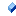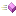The following tables list the members exposed by TAgkPoint.

 Name DescriptionX-coordinate of the point.Y-coordinate of the point.
 Name DescriptionThis is the overview for the Clamp method overload.This is the overview for the Create method overload.This is the overview for the CrossProduct method overload.This is the overview for the Distance method overload.This is the overview for the DistanceSq method overload.This is the overview for the DotProduct method overload.Checks whether this point equals another point.Calculates the unit length vector for the given angle (in degrees).Calculates the unit length vector for the given angle (in radians).This is the overview for the Length method overload.This is the overview for the LengthSq method overload.This is the overview for the LinearInterpolate method overload.This is the overview for the LinearInterpolateConst method overload.This is the overview for the Near method overload.This is the overview for the Normalize method overload.This is the overview for the NormalizeSafe method overload.This is the overview for the Perp method overload.This is the overview for the Project method overload.This is the overview for the Rotate method overload.This is the overview for the RPerp method overload.This is the overview for the ToAngle method overload.This is the overview for the ToAngleRad method overload.This is the overview for the Unrotate method overload.
 Name DescriptionAdd two points (or vectors).Checks if two points are equal.This is the overview for the Multiply operator overload.Negate a point (or vector).Checks if two points are not equal.Subtracts two points (or vectors).
 Copyright (c) 2012. All rights reserved.